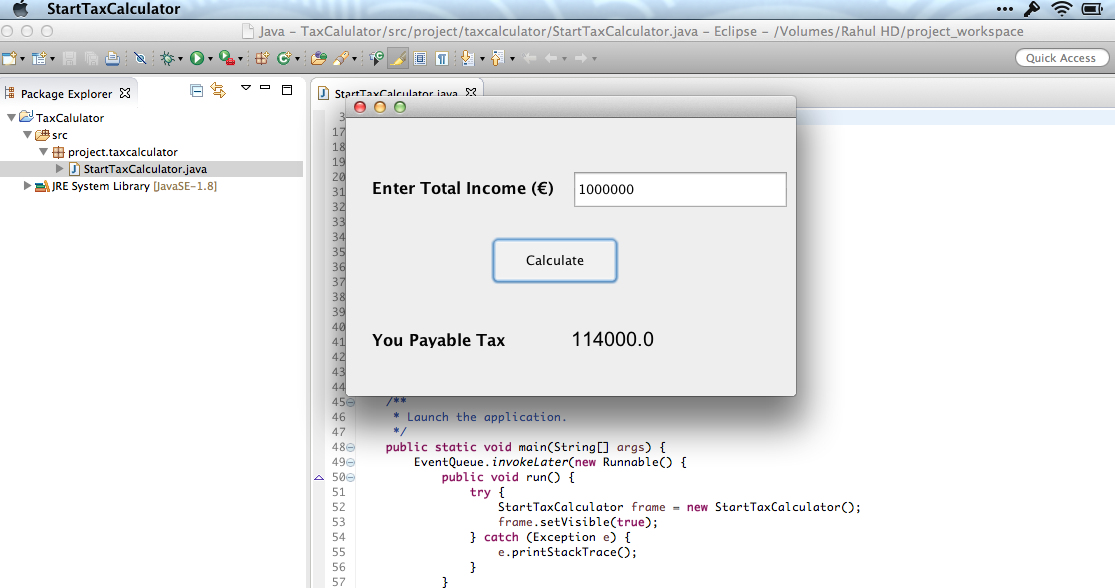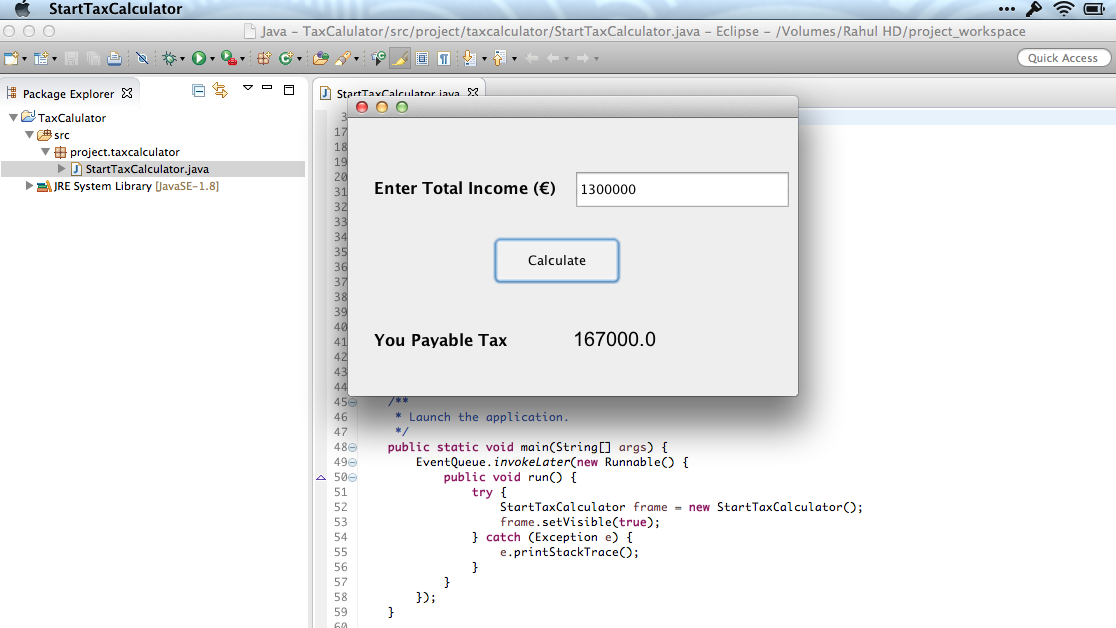## Welcome to the Treehouse Community

Want to collaborate on code errors? Have bugs you need feedback on? Looking for an extra set of eyes on your latest project? Get support with fellow developers, designers, and programmers of all backgrounds and skill levels here with the Treehouse Community!

### Looking to learn something new?

Treehouse offers a seven day free trial for new students. Get access to thousands of hours of content and join thousands of Treehouse students and alumni in the community today.# Percentage income problem

Hi Everyone,

I'm trying to write a program to make a simple tax calculator . My problem is breaking down the calculations into steps using loops and conditional statements, I'll appreciate it if you guys can help me out.

Tax Due is computed at the following applicable rates:

1st 300000 of taxable Income at 7%

Next 300000 11%

Next 500000 15%

Next 500000 19%

Next 1600000 21%

Above 3200000 24%

Thanks and hope to here from you soon.

Hi Stephen,

Just picked up your question on a quiet Sunday evening. I wrote a small program for a simple GUI based tax calculator Complete project on Github

Sharing below an excerpt from the main project that lays down the logic

```// Checking condition for 1st 300000 of taxable income at 7%
if(totalIncome <= 300000){
taxValue = totalIncome * 0.07;
}
else if (totalIncome > 300000){
taxValue = 300000 * 0.07;
}

totalIncome = totalIncome - 300000;

// Checking condition for next 300000 of taxable income at 11%
if(totalIncome == 0 || totalIncome < 0){
return taxValue;
}
else if (totalIncome<300000 && !(totalIncome < 0)){
taxValue = taxValue + (totalIncome * 0.11);
}
else if (totalIncome > 300000){
taxValue = taxValue + (300000*0.11);
}

totalIncome = totalIncome - 300000;

// ... and so on for other conditions
```

So basically this is what the algorithm looks like

1) Input total income

2) Set total tax value to 0

3) Check first condition

3.1) If total income is less than or equal to 300000 then tax value is equal to 7% of given income. Return the tax result
3.2) Else if income is greater than 300000 then tax value is equal to 7% of 300000. Return the tax result
3.3) Subtract 300000 from the total income

4) Check next condition

4.1) If total income is equal to zero or a negative value return the perviously calculated tax result

4.2) Else if total income after deduction in 3.3 is less than 300000 and not a negative value then tax value is equal to tax value calculated in 3.2 + 11% of remaining income. Return the tax result

4.3) Else if income is greater than 300000 then tax value is equal to tax value calculated in 3.2 + 11% of 300000 . Return tax value result

4.4) Subtract 300000 again from the total income

5) Repeat steps above for other conditions

Again this is a casual attempt and there could be other easier ways to do this though I'm always open for suggestions.

Hope this helps.I tried out your code but its not working as it should, its failing with big amount like 1,000,000 and above. Please look at Chris Hall's explanation, I think he has a better understanding of what I want to achieve. Thanks

Hi Stephen,

Well as I said, it was just a casual attempt. There could be errors. However I was just checking it again and it seems to be working fine for values 1000000 and above. I've displayed the screen shotsYes, it will because after 3200000 I did not write a condition for it. So any value above 32,000,00 will give you the same answer. It was only to set a template to work on. Just add more conditions after the value 32,00,000 and it should give you the desired resultsHi Stephen, First I would figure out which tax bracket the taxable income would fall under. For each tax bracket, you can calculate the total tax at that percentage if the taxable income is equal or greater.

For example:

300,000 * 0.07 = 21,000

300,000 * 0.11 = 33,000

500,000 * 0.15 = 75,000

Then what you would do is for every previous tax bracket, subtract the bracket amount from the taxable income. Then calculate the tax on the remainder for the current tax bracket.

Another example with 1,000,000 taxable income (highest tax bracket 15%)

1,000,000 - 300,000 = 700,000 || tax = 21,000

700,000 - 300,000 = 400,000 || tax = 33,000 + 21,000

400,000 * 0.15 = 60,000 || tax = 60,000 + 33,000 + 21,000

Total tax of 114,000 for 1,000,000 taxable income.